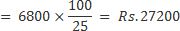## Profit and Loss For SBI PO Set – 2

1) A salesman sells shirts at a profit of 10%. When he increases the selling price by Rs.25 per shirt, the profit increases by 5%. Find the new S.P

a) Rs.500

b) Rs.525

c) Rs.575

d) Cannot be determined

c) Rs.575

Explanation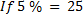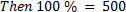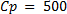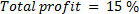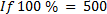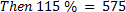2) Babli bought 30 pens and 10 pencils for Rs.600. She sold the pens at a profit of 10% and pencils at a profit of 20% and made a total profit of Rs.90. Find the cost price per pen.

a) Rs.15

b) Rs.5

c) Rs. 20

d) Rs. 10

e) None of these

d) Rs. 10

Explanation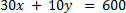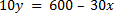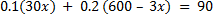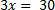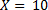3) A cloth merchant, selling at 15% profit uses a faulty measuring rod that measures 90cm for a metre. Find his profit percent actually earned

a) 17%

b) 37.5%

c) 27.7%

d) 21.2%

e) None of these

c) 27.7%

Explanation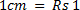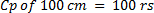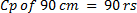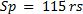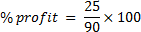4) The cost price of two TV is equal. One TV is sold at a profit of 30% and the other one for 5504 less than the first one. If the overall profit earned after selling both of TV is 14%, what is the cost price of each TV?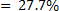a) 20,313

b) 20,314

c) 20,315

d) 24,444

e) 17,200

e) 17,200

Explanation

Let the cost price of each TV is x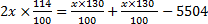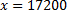5) A milkman buys some milk. If he sells it at rupees 10 per litre, he losses 400 rupees but when he sells it at 12 per litre, he gains 800 rupees. How much milk did he purchase?

a) 400 litre

b) 550 litre

c) 600 litre

d) 800 litre

e) None of these

c) 600 litre

Explanation

10x=s-400

12x=s+800

2x=1200

X=600

6) A vendor sold two magazines namely A and B. He sold magazine ‘A’ at a loss of 30% and magazine ‘B’ at a profit of 35% but finally there is no loss or no gain. If the total Selling price of both magazines is Rs.572. Find the difference between the Cost Price of Magazine ‘A’ and ‘B’?

a) Rs. 32

b) Rs. 44

c) Rs. 56

d) Rs. 62

e) None of the Above

b) Rs. 44

Explanation

30% of x = 35% of y

x + y = 572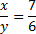Difference = Rs.44

7) On a Rs. 10,000 payment order, a person has choice between 3 successive discounts of 10%, 10% and 30%, and 3 successive discounts of 40%, 5% and 5%. By choosing the better one he can save (in Rupees) ?

a) 200

b) 255

c) 400

d) 433

e) 325

b) 255

Explanation

= 70% of 90% of 90% of Rs. 10000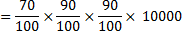= Rs. 5670

Selling price in the second case

= 95% of 95% of 60% of Rs. 10000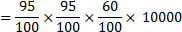= Rs. 5415

Saving = Rs. (5670 – 5415) = Rs. 255

8) A shopkeeper purchased some goods for Rs.900and sold one third of the goods at a loss of 12%, then at what percent at gain should the remainder goods he sold  to gain 18% profit on the whole transaction?

a) 31%

b) 26%

c) 33%

d) 18%

e) None of these

c) 33%

Explanation

To gain 18% profit he should sell the goods at= (900×118)/100=1062

At  rate of 12% loss the goods are sold at= 1062-(300×88)/100=798

The remainimg parts are worth of rs.600 which is sold at= 798-600=198

So the rate at which he should sell the goods= (198/600)×100=33%

9) A shopkeeper bought 30 kg of wheat at the rate of Rs. 45 per kg. He sold 40% of the total quantity at the rate of Rs. 50 per kg. Approximately, at what price per kg should he sell the remaining quantity to make 25 percent overall profit?

a) Rs.54

b) Rs.52

c) Rs.50

d) Rs.60

e) Rs.56

d) Rs.60

Explanation

C.P of wheat = 30×45=1350

40% of 30kg = 12 kg

S.P of 12 kg =12 ×50=Rs.600

25% profit of, total S.P of all the wheat is Rs.1687.5

Remaining Wheat (30-12) =18 kg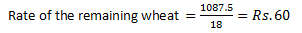10) Prashant incurred a loss of 75 percent on selling an article for Rs. 6,800. What was the cost price of the article?

a) Rs. 27,700

b) Rs. 25,600

c) Rs. 21,250

d) Rs. 29,000

e) None of these

e) None of these

Explanation

C.P of article